# Some microsynth notes

My question is from our CPP project on business improvement districts (Piza, Wheeler, Connealy, Feng 2020). The article indicates that you ran three of the microsynth matching variables as an average over each instead of the cumulative sum (street length, percent new housing structures, percent occupied structures). How did you get R to read the variables as averages instead of the entire sum of the treatment period of interest? I have the microsynth code you used to generate our models, but cannot seem to determine how you got R to read the variables as averages.

So Nate is talking about this paper, Crime control effects of a police substation within a business improvement district: A quasi-experimental synthetic control evaluation (Piza et al., 2020), and here is the balance table in the paper: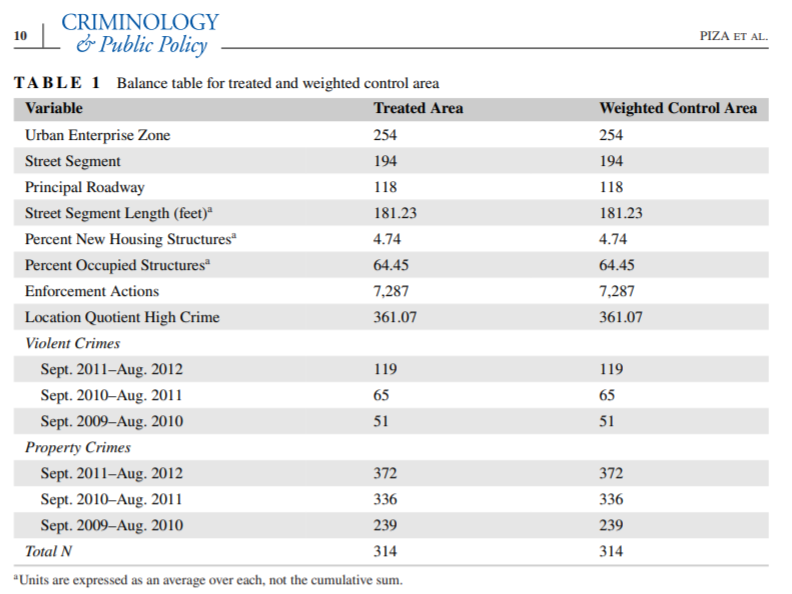To be clear to folks, I did not balance on the averages, but simply reported the table in terms of averages. So here is the original readout from R: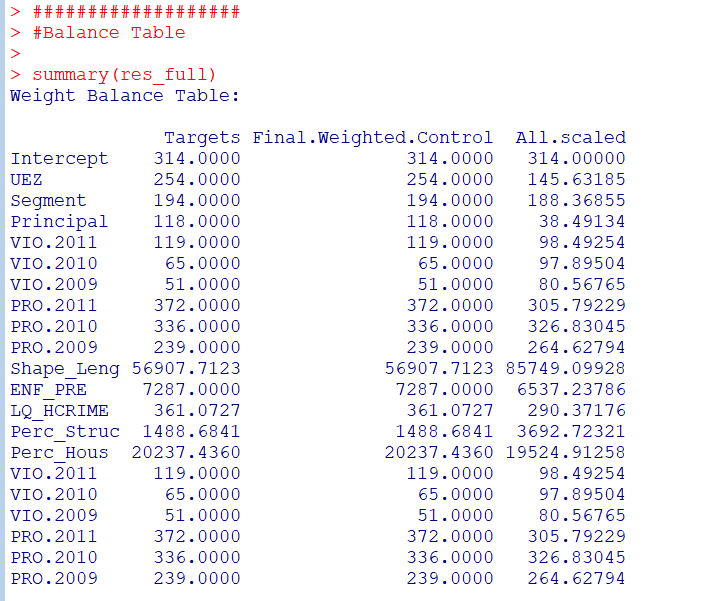So I just divided those noted rows by 314 to make them easier to read. You could divide values by the total number of treated units though in the original data to have microsynth match on the averages instead if you wanted to. Example below (this is R code, see the microsynth library and paper by Robbins et al., 2017):

``````library(microsynth)
set.seed(10)

data(seattledmi) #just using data in the package
cs <- seattledmi
# calculating proportions
cs\$BlackPerc <- (cs\$BLACK/cs\$TotalPop)*100
cs\$FHHPerc <- (cs\$FEMALE_HOU/cs\$HOUSEHOLDS)*100
# replacing 0 pop with 0
cs[is.na(cs)] <- 0

cov.var <- c("TotalPop","HISPANIC","Males_1521","FHHPerc","BlackPerc")
match.out <- c("i_felony", "i_misdemea")

sea_prop <- microsynth(cs,
idvar="ID", timevar="time", intvar="Intervention",
start.pre=1, end.pre=12, end.post=16,
match.out.min=match.out,match.out=FALSE,
match.covar=FALSE,check.feas=FALSE,
match.covar.min=cov.var,
result.var=match.out)

summary(sea_prop) # balance table``````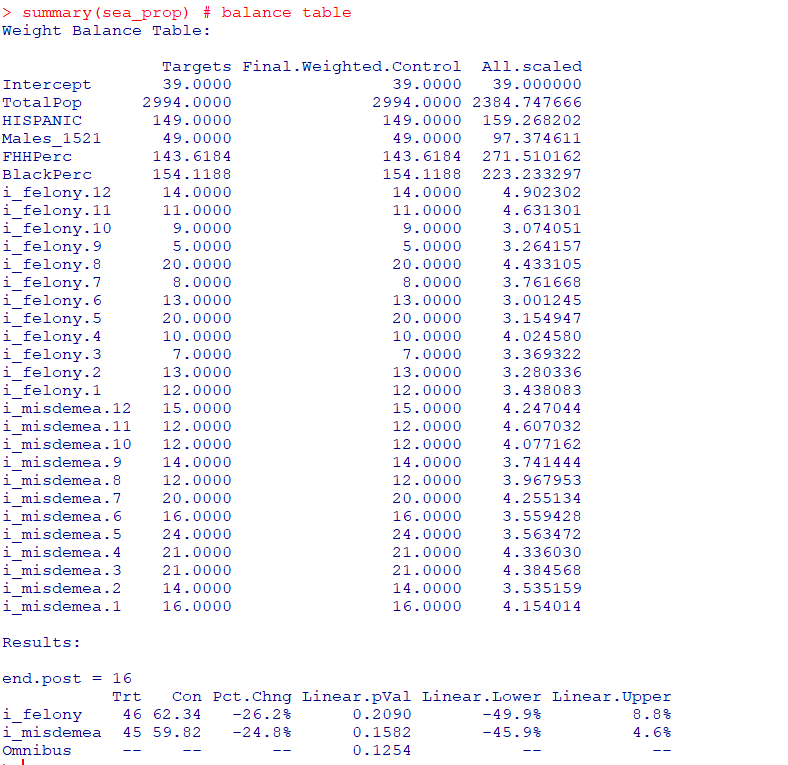And here you can see that we are matching on the cumulative sums for each of the areas, but we can divide our covariates by the number of treated units, and we will match on the proportional values.

``````# Can divide by 39 and get the same results
cs[,cov.var] <- cs[,cov.var]/39

sea_div <- microsynth(cs,
idvar="ID", timevar="time", intvar="Intervention",
start.pre=1, end.pre=12, end.post=16,
match.out.min=match.out,match.out=FALSE,
match.covar=FALSE,check.feas=FALSE,
match.covar.min=cov.var,
result.var=match.out)

summary(sea_div) # balance table``````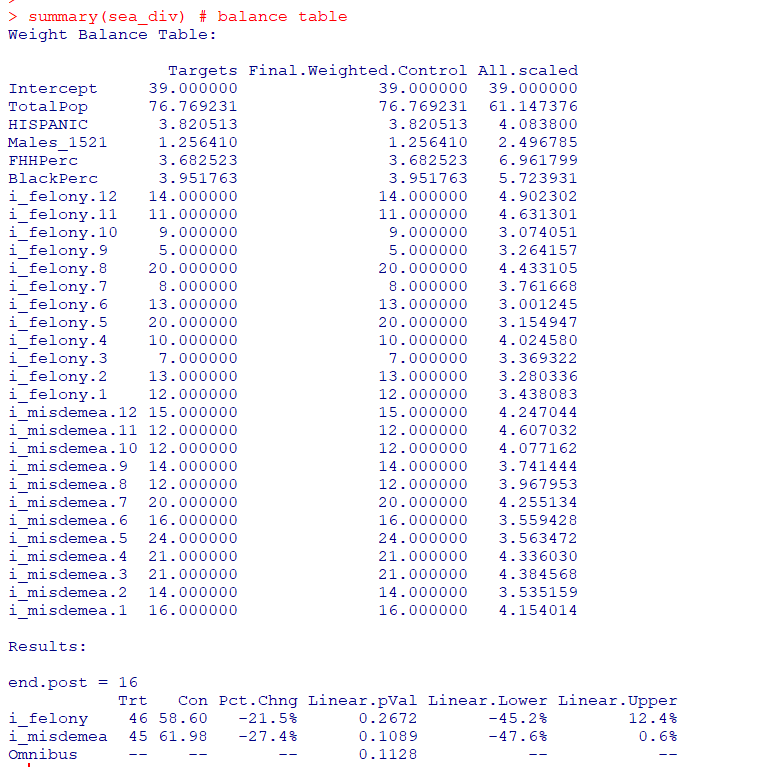Note that these do not result in the same weights. If you look at the results you will see the treatment effects are slightly different. Also if you do:

``````# Showing weights are not equal
all.equal(sea_div\$w\$Weights,sea_prop\$w\$Weights)``````

It does not return True. Honestly not familiar enough with the procedure that microsynth uses to do the matching (Raking survey weights) to know if this is due to stochastic stuff or due to how the weighting algorithm works (I would have thought a linear change does not make a difference, but I was wrong).

On the bucket list is to do a matching algorithm that returns geographically contiguous areas and gives the weights all values of 1 (so creates comparable neighborhoods), instead of estimating Raking weights. That may be 5 years though before I get around to that. Gio has a nice map to show the way the weights work now is they may be all over the place (Circo et al., 2021) – I am not sure that is a good thing though.

But I did want to share some functions I used for the paper I worked with Nate on. First, this is for if you use the permutation approach, the function `prep_synth` returns some of the data in a nicer format to make graphs and calculate your own stats:

``````# Function to scoop up the data nicely
prep_synth <- function(mod){
#Grab the plot data
plotStats <- mod[['Plot.Stats']]
#For the left graph
Treat <- as.data.frame(t(plotStats\$Treatment))
Treat\$Type <- "Treat"
#This works for my data at years, will not
#Be right for data with more granular time though
Treat\$Year <- as.integer(rownames(Treat))
Cont <- as.data.frame(t(plotStats\$Control))
Cont\$Type <- "Control"
Cont\$Year <- as.integer(rownames(Cont))
AllRes <- rbind(Treat,Cont)
#For the right graph
Perm <- as.data.frame(t(as.data.frame(plotStats\$Difference)))
SplitStr <- t(as.data.frame(strsplit(rownames(Perm),"[.]")))
colnames(SplitStr) <- c("Type","Year")
rownames(SplitStr) <- 1:nrow(SplitStr)
SplitStr <- as.data.frame(SplitStr)
Perm\$Type <- as.character(SplitStr\$Type)
Perm\$Year <- as.integer(as.character(SplitStr\$Year))
Perm\$Group <- ifelse(Perm\$Type == 'Main','Treatment Effect','Permutations')
#Reordering factor levels for plots
AllRes\$Type <- factor(AllRes\$Type,levels=c('Treat','Control'))
levels(AllRes\$Type) <- c('Treated','Synthetic Control')
Perm\$Group <- factor(Perm\$Group,levels=c('Treatment Effect','Permutations'))
#Exporting result
Res <- vector("list",length=2)
Res[] <- AllRes
Res[] <- Perm
names(Res) <- c("AggOutcomes","DiffPerms")
return(Res)
}``````

It works for the prior tables, but I really made these functions to work with when you used permutations to get the errors. (In the micro synth example, it is easier to work with permutations than in the state level example for synth, in which I think conformal prediction intervals makes more sense, see De Biasi & Circo, 2021 for a recent real example with micro place based data though.)

``````# Takes like 1.5 minutes
sea_perm <- microsynth(seattledmi,
idvar="ID", timevar="time", intvar="Intervention",
start.pre=1, end.pre=12, end.post=16,
match.out.min=match.out,match.out=FALSE,
match.covar=FALSE,check.feas=FALSE,
match.covar.min=cov.var,
result.var=match.out, perm=99)

res_prop <- prep_synth(sea_perm)
print(res_prop)``````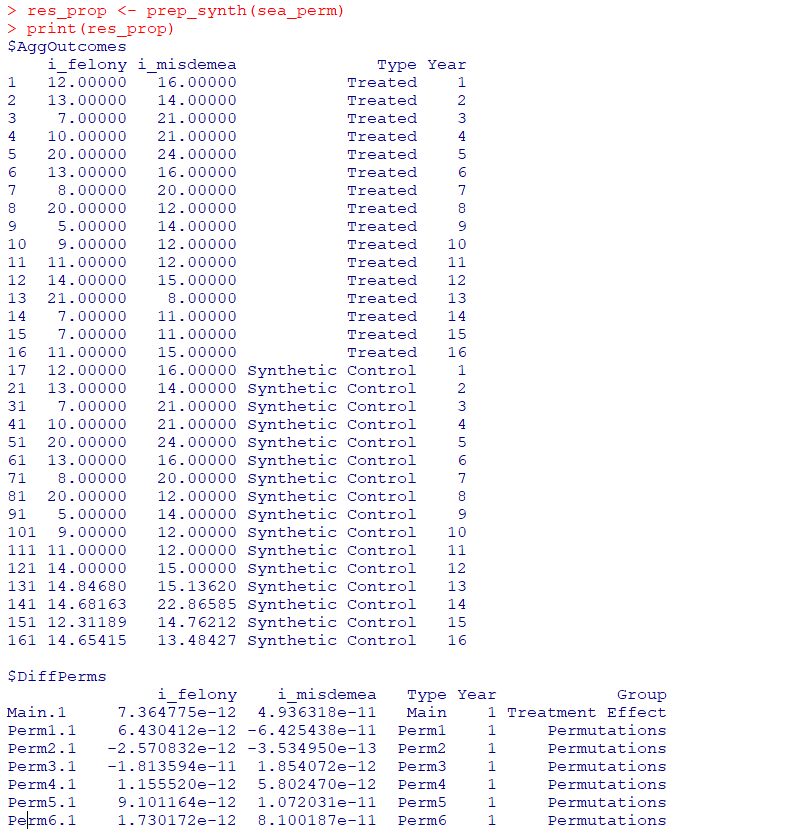So the dataframe in the first slot is the overall treatment effect, and the second dataframe is a nice stacked version for the permutations. First, I really do not like the percentage change (see Wheeler, 2016 for the most direct critique, but I have a bunch on this site). So I wrote code to translate the treatment effects into crime count reductions instead of the percent change stuff.

``````# Getting the observed treatment effect on count scale
# vs the permutations

agg_fun <- function(x){
sdx <- sd(x)
minval <- min(x)
l_025 <- quantile(x, probs=0.025)
u_975 <- quantile(x, probs=0.975)
maxval <- max(x)
totn <- length(x)
res <- c(sdx,minval,l_025,u_975,maxval,totn)
return(res)
}

treat_count <- function(rp){
# Calculating the treatment effect based on permutations
keep_vars <- !( names(rp[]) %in% c("Year","Group") )
out_names <- names(rp[])[keep_vars][1:(sum(keep_vars)-1)]
loc_dat <- rp[][,keep_vars]
agg_treat <- aggregate(. ~ Type, data = loc_dat, FUN=sum)
n_cols <- 2:dim(agg_treat)
n_rows <- 2:nrow(agg_treat)
dif <- agg_treat[rep(1,max(n_rows)-1),n_cols] - agg_treat[n_rows,n_cols]
dif\$Const <- 1
stats <- aggregate(. ~ Const, data = dif, FUN=agg_fun)
v_names <- c("se","min","low025","up975","max","totperm")
long_stats <- reshape(stats,direction='long',idvar = "Const",
varying=list(2:ncol(stats)),
v.names=v_names, times=out_names)
# Add back in the original stats
long_stats <- long_stats[,v_names]
rownames(long_stats) <- 1:nrow(long_stats)
long_stats\$observed <- t(agg_treat[1,n_cols])[,1]
long_stats\$outcome <- out_names
ord_vars <- c('outcome','observed',v_names)
return(long_stats[,ord_vars])
}

treat_count(res_prop)``````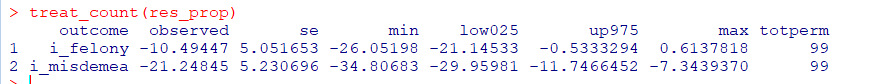So that is the cumulative total effect of the intervention. This is more similar to the WDD test (Wheeler & Ratcliffe, 2018), but since the pre-time period is matched perfectly, just is the differences in the post time periods. And here it uses the permutations to estimate the error, not any Poisson approximation.

But I often see folks concerned about the effects further out in time for synthetic control studies. So here is a graph that just looks at the instant effects for each time period, showing the difference via the permutation lines:

``````# GGPLOT graphs, individual lines
library(ggplot2)
perm_data <- res_prop[]
# Ordering factors to get the treated line on top
perm_data\$Group <- factor(perm_data\$Group, c("Permutations","Treatment Effect"))
perm_data\$Type <- factor(perm_data\$Type, rev(unique(perm_data\$Type)))
pro_perm <- ggplot(data=perm_data,aes(x=Year,y=i_felony,group=Type,color=Group,size=Group)) +
geom_line() +
scale_color_manual(values=c('grey','red')) + scale_size_manual(values=c(0.5,2)) +
geom_vline(xintercept=12) + theme_bw() +
labs(x=NULL,y='Felony Difference from Control') +
scale_x_continuous(minor_breaks=NULL, breaks=1:16) +
scale_y_continuous(breaks=seq(-10,10,2), minor_breaks=NULL) +
theme(panel.grid.major = element_line(linetype="dashed"), legend.title= element_blank(),
legend.position = c(0.2,0.8), legend.background = element_rect(linetype="solid", color="black")) +
theme(text = element_text(size=16), axis.title.y=element_text(margin=margin(0,10,0,0)))``````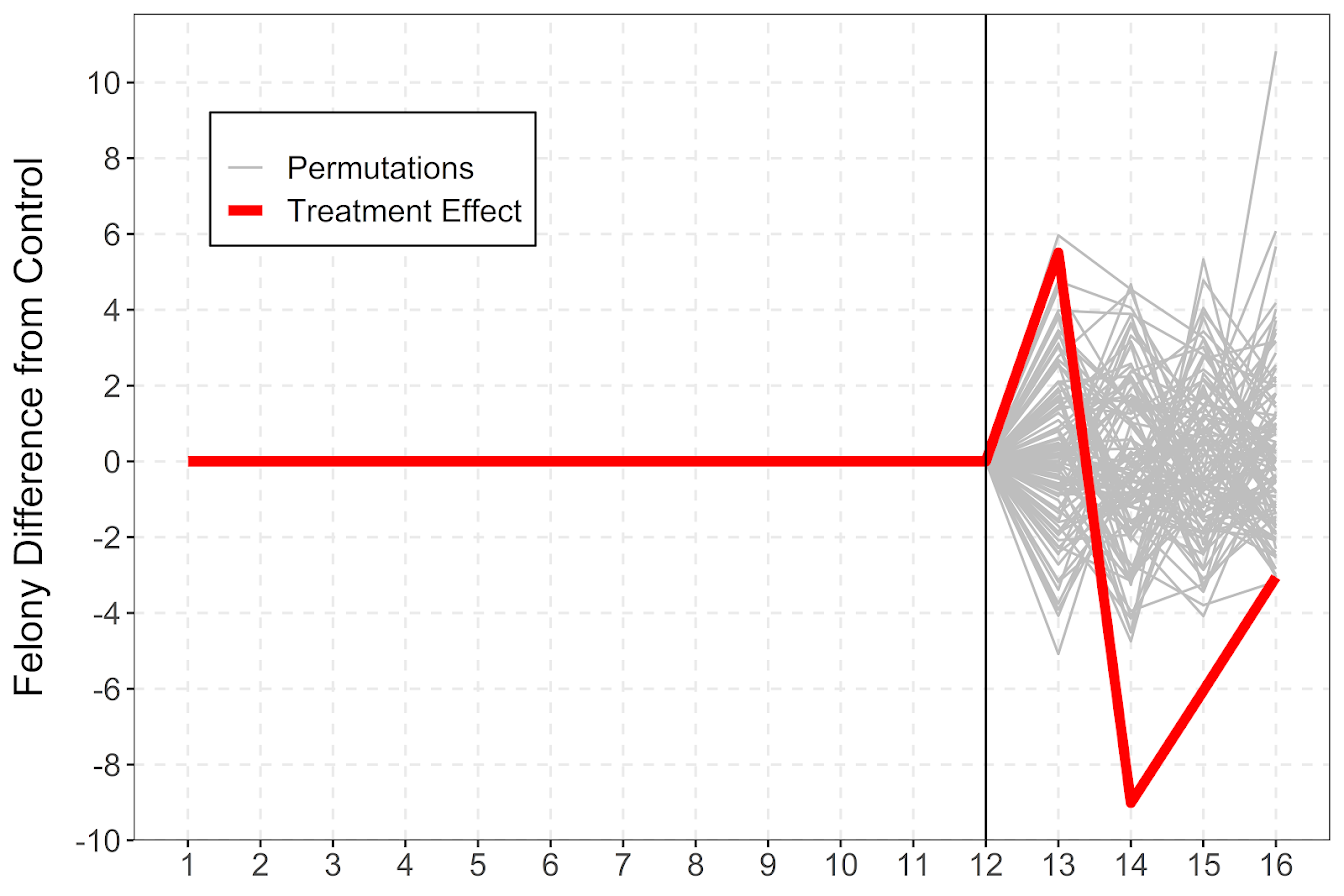And I also like looking at this for the cumulative effects as well, which you can see with the permutation lines widen over time.

``````# Cumulative vs Pointwise
perm_data\$csum_felony <- ave(perm_data\$i_felony, perm_data\$Type, FUN=cumsum)
pro_cum  <- ggplot(data=perm_data,aes(x=Year,y=csum_felony,group=Type,color=Group,size=Group)) +
geom_line() +
scale_color_manual(values=c('grey','red')) + scale_size_manual(values=c(0.5,2)) +
geom_vline(xintercept=12) + theme_bw() +
labs(x=NULL,y='Felony Difference from Control Cumulative') +
scale_x_continuous(minor_breaks=NULL, breaks=1:16) +
scale_y_continuous(breaks=seq(-20,20,5), minor_breaks=NULL) +
theme(panel.grid.major = element_line(linetype="dashed"), legend.title= element_blank(),
legend.position = c(0.2,0.8), legend.background = element_rect(linetype="solid", color="black")) +
theme(text = element_text(size=16), axis.title.y=element_text(margin=margin(0,10,0,0)))``````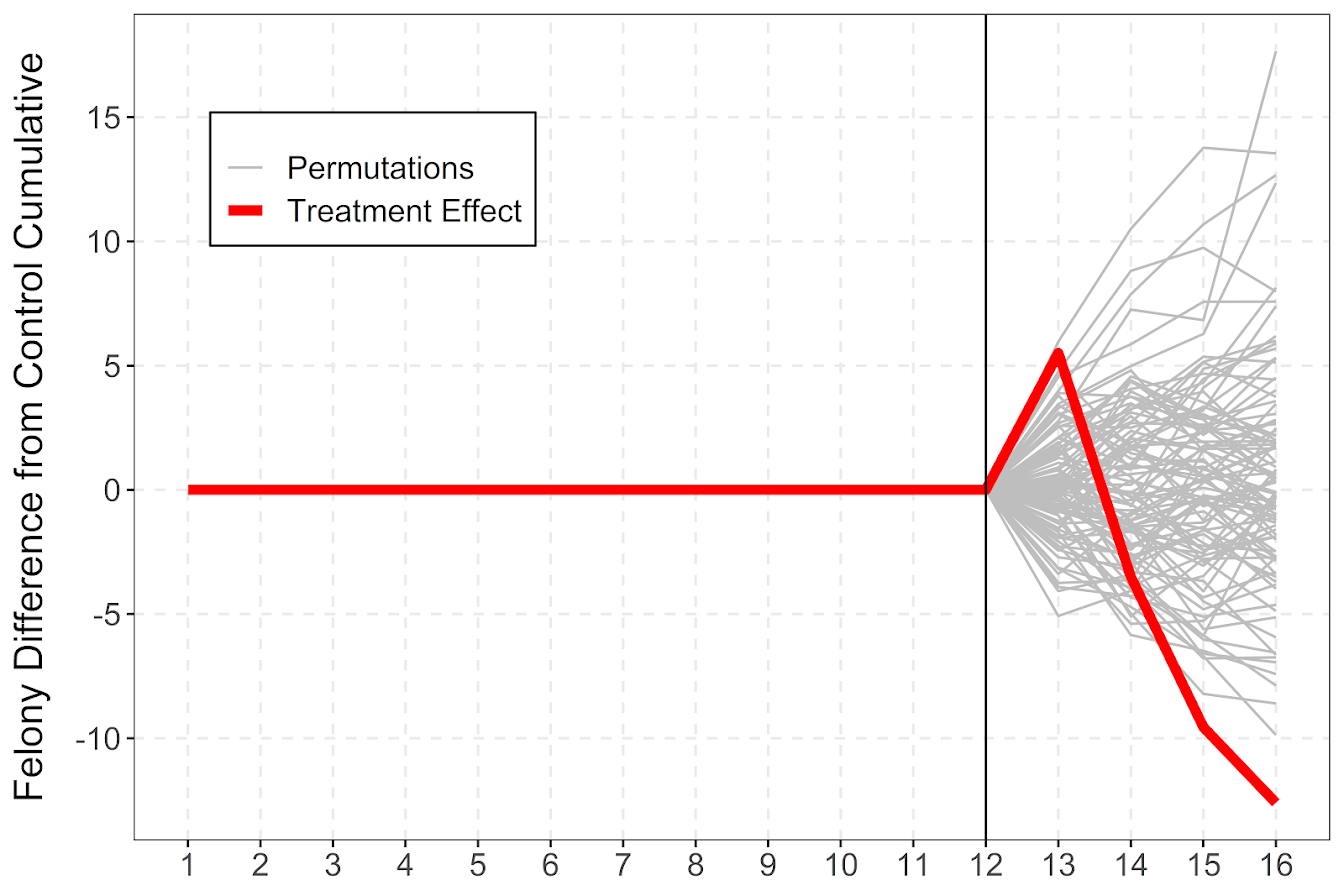If you do a ton of permutations (say 999 instead of 99), it would likely make more sense to do a fan chart type error bars and show areas of different percentiles instead of each individual line (Yim et al., 2020).

I will need to slate a totally different blog post to discuss instant vs cumulative effects for time series analysis. Been peer-reviewing quite a few time series analyses of Covid and crime changes – most everyone only focuses on instant changes, and does not calculate cumulative changes. See for example estimating excess deaths for the Texas winter storm power outage (Aldhous et al., 2021). Folks could do similar analyses for short term crime interventions. Jerry has a good example of using the Causal Impact package to estimate cumulative effects for a gang takedown intervention (Ratcliffe et al., 2017) for one criminal justice example I am familiar with.

Again for folks feel free to ask me anything. I may not always be able to do as deep a dive as this, but always feel free to reach out.

1.#### Robert

/  May 16, 2022

Hi Andrew,

Just a quick question for you! From the case study you performed a microsynth I understand that we have two causal relationships we want to discuss.

The first is: i_felony = TotalPop + HISPANIC + Males_1521 + FHHPerc+BlackPerc & the second is: i_misdemea= TotalPop + HISPANIC + Males_1521 + FHHPerc+BlackPerc

How would we go about obtaining the outcome point estimates that a typical regression would output? Would they be just running your 3rd last code chuck and thus the estimates for the outcome variables i_felony and i_misdemea be -10.49 and -21.24?

Thanks!

•#### apwheele

/  May 16, 2022

Not exactly sure what your question is Robert. The point estimates you noted are:

Observed – E[Crime]

where E[Crime], “expected crime”, is estimated via the synthetic control areas (constructed from demographics + pre-intervention trends). And the standard errors are estimated via a permutation approach, where we shuffle what micro areas are assigned treatment, redo the whole analysis, and see how much E[Crime] varies over the permutations.

When you say “point estimates that a typical regression would output”, I am thinking about coefficients on the right hand side. This isn’t quite the same, the demographics are matched to be 100% the same for this sample.

You might check out this other blog post I did, showing how to use lasso regression to estimate synthetic control, https://andrewpwheeler.com/2019/12/06/using-regularization-to-generate-synthetic-controls-and-conformal-prediction-for-significance-tests/, to see how the weights can be related to regression. They are not a 100% map to one another though.

•#### Robert

/  May 16, 2022

Thanks Andrew, perhaps the better question to ask is how can we see (calculate) how much of an effect do our “match.out” variables (i_felony & i_misdemea) influence the E[crime].

Similar to how the coefficients from the RHS of a regression influence the outcome variable.

•#### apwheele

/  May 16, 2022

i_felony and i_misdemea are the *outcome* variables on the left hand side though — so I don’t understand the question. They define E[crime].